top

Home ] workshop ] how does it work ] History of Astronautics page index ] Rocket launches ] useful sites ] version francaise ] Contact us ]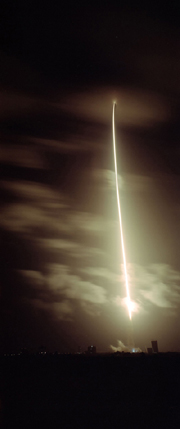Ariane 5   Kourou

 Specific Impulse Specific impulse is the parameter used to measure the efficiency of a rocket jet engine, and is basically the ratio of the useful jet impulse Ft (Ns) produced divided by  the fuel  flow-rate (mass per second in Kg/sec) required to produce that impulse . Specific impulse Isp is the index of performance for rocket engines and is defined by the following ratio :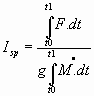sec Where the numerator is the total impulse obtained over the operating time of the rocket engine. Usually referred to as the ‘Burn time’. Defined by the time period from t0 to t1.seconds. Accompanied by a denominator which represents the total fuel mass flow necessary to produce the impulse during the same time period. ‘g’ is the acceleration due to gravity gearth at sea level m/s2 The above expression can be simplified to the ratio given below if the flow rate and thrust are constant over the burn time. For liquid fueled rocket engines this is usually the case,  so they can be represented  by the ratio of the thrust force produced  at the exit of the nozzle F    divided by the product of  gearth  at sea level  multiplied by the mass flow rate of fuel used to produce the thrust. Note: F is measured at  tearth Specfic  impulse       Isp =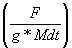sec Where F is the Jet Thrust force at the outlet of the nozzle F = q * v2         N   Newtons    ‘q’             is the volumetric flowrate * density r  to give mass flow-rate of fluid leaving the nozzle Kgs/sec v2               is the exhaust jet velocity at the outlet of the nozzle m/sec Mdt          is the mass flowrate of fuel Dimensional analysis of Isp Isp  =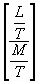Sec Isp =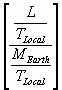Sec Research Page esa comparison of specific impulse for liquid propergols and electric propulsion  Once you are out in space and have overcome aerodynamic drag of the atmosphere then the total impulse produced over a long period by a certain mass of fuel become significant.    http://sci.esa.int/science-e/www/object/index.cfm?fobjectid=34201 Definition of Tsiolkovskis Formula Tsiolkovski modified end function Top http://en.wikipedia.org/wiki/Specific_impulse   © All rights reserved by John Gwynn                                                                Water Rocket Explorer [ back ] [ menu of this section ] [ next ] 12/01/07 This site was created on the 15th April 2003  ©John Gwynn and sons2003  You're welcome to reproduce any material on this site for educational or other non commercial purposes  as long as you give us proper credit (by referring to "The Water-Rocket Explorer" http://waterocket.explorer.free.fr).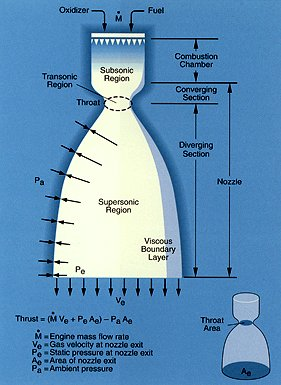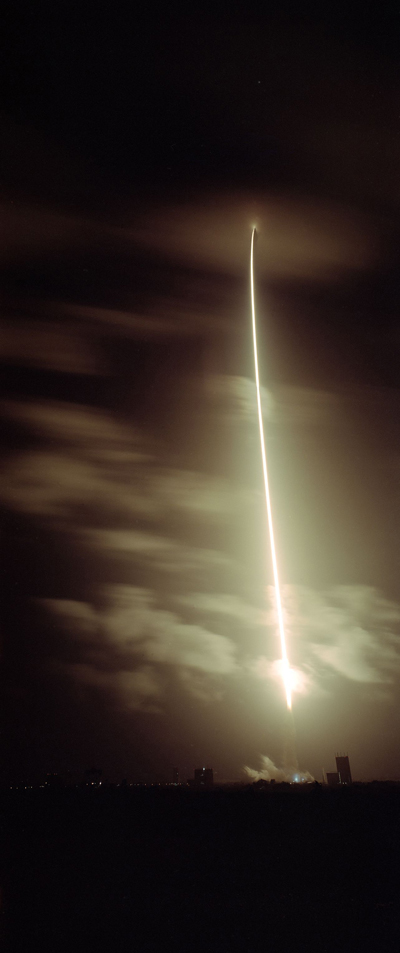Ariane 5 Envisat 2 " Great things are not done by impulse, but a series of small things brought together". Vincent Van Gogh Ironic quotation which says that success is the result of assembling many  simple existng systems or ideas in a new original way.